# AP Calculus AB Practice Test 7

### Test Information10 questions20 minutes

Calculator Disallowed

1.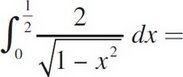2. Find a positive value c, for x, that satisfies the conclusion of the Mean Value Theorem for Derivatives for f(x) = 3x2 - 5x + 1 on the interval [2, 5].

3. Given f(x) = 2x2 - 7x - 10, find the absolute maximum of f(x) on [-1, 3].

4. Find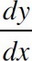If x3y + xy3 = -10.

5.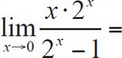6.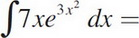7. Find the equation of the tangent line to 9x2 + 16y2 = 52 through (2, -1).

8. A particle's position is given by s = t3 - 6t2 + 9t. What is its acceleration at time t = 4 ?

9. If f(x) = 3πx, then f′(x) =

10. The average value of f(x) =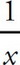from x = 1 to x = e is